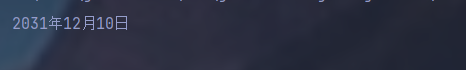﻿ JavaSE系列基础包装类及日历类详解_java_脚本之家
java# JavaSE系列基础包装类及日历类详解

## 1. 基本类型包装类

### 1.1 概述```public class IntegerDemo {
public static void main(String[] args) {
//判断数据是否在int范围内
System.out.println(Integer.MAX_VALUE);//最大范围
System.out.println(Integer.MIN_VALUE);//最小范围
}
}
```

### 1.2 Integer类的概述与使用```Integer i3=new Integer("asd");//这里就会运行错误
```

```public class IntegerDemo {
public static void main(String[] args) {
Integer i1=Integer.valueOf(123);//数字类型
System.out.println(i1);
Integer i2=Integer.valueOf("123");//字符串类型
}
}

```

### 1.3 int和String的相互转换

```int number=100;
String s1=""+number;
```String—>Integer—>int

```public class IntegerDemo {
public static void main(String[] args) {
int num=100;
String s1=String.valueOf(num);
//int转换为字符串类型
System.out.println(s1);
System.out.println("----------");
//字符串转换为int
String s2="100";
// 方式一:
//String--->Integer--->int
Integer i1=Integer.valueOf(s2);
//通过帮助文档发现，
// intValue()用来返回Integer
// 作为int的值，正好符合要求
int x=i1.intValue();//返回值用int接收
System.out.println(x);
//方式二：使用parseInt静态方法
int y=Integer.parseInt(s2);
System.out.println(y);
}
}
```

### 1.4 案例-字符串中数据排序```public class IntegerDemo {
public static void main(String[] args) {
//定义字符串
String s="91 27 46 38 56";
//将字符串中数据抽离出来,以空格作为分割点
String[] s1 = s.split(" ");
//定义一个int数组，用于存这些数据
int []arr=new int[s1.length];//以s1的长度
//转换为int类型
for (int i = 0; i < s1.length; i++) {
arr[i] = Integer.parseInt(s1[i]);
//赋值
}
//进行排序
Arrays.sort(arr);
//字符串拼拼接
StringBuilder sb=new StringBuilder();
for(int i = 0; i < arr.length; i++){
if(i==arr.length-1){
sb.append(arr[i]);
}else{
sb.append(arr[i]).append(" ");
}

}
String result = sb.toString();
System.out.println("排序后的："+ result);

}
}
```

### 1.5 自动装箱和拆箱

```Inter i=Integer.valueOf(100);//这里先把100转化为Inter类型，赋值
Integer i1=100;//这里java5之后就在jdk内部
//已经潜移默化的为我们包装好了
//它等同于上步
```

```Integer i1=100;
//拆箱：转换为int型，用intValue()这个方法
//然后进行基本类型的运算后再赋值给i1
//完成自动拆箱
i1=i1.intValue()+200;//最后赋值是自动装箱
```

```Integer i1=100;
i1+=200;//内部隐含了自动拆箱和自动装箱
```

## 2. Date类Date 代表了指定时间，精确到毫秒```public class DateDemo {
public static void main(String[] args) {
Date d=new Date();
//按道理输出的应该是地址，结果输出了时间，因为重写了toString方法
System.out.println(d);
//有参构造
long data=1000*60*60;//毫秒*1000->秒->*60->分钟->*60->小时
Date d2=new Date(data);
System.out.println(d2);
}
}
```

Wed Dec 15 17:51:04 CST 2021

Thu Jan 01 09:00:00 CST 1970//其中09:00:00是时区

### 2.1 Date常用方法

public long getTime() 获取的是日期对象，从1970年1月1日00：00：00到现在的毫秒

public void setTime(long time) 设置时间，给的是毫秒值

```getTime:
public class DateDemo {
public static void main(String[] args) {
Date d=new Date();
//获取时间
System.out.println(d.getTime()*1.0/1000/60/60/24/365+"年");
}
}

```

51.99024939979706年//从1970到现在

```setTime
public class DateDemo {
public static void main(String[] args) {
Date d=new Date();
long date=1000*60*60;
d.setTime(date);
System.out.println(d);
}
}
```

Thu Jan 01 09:00:00 CST 1970

## 3. SimpleDateFormat类

SimpleDateFormat是一个具体的类，用于以区域设置敏感的方式格式化和解析日期。重点学习日期格式化和解析化

• y…年
• M…月
• d…天
• H…时
• m…分
• s…秒SimpleDateFormat格式化与解析日期

```import java.text.SimpleDateFormat;
import java.util.Date;

public class SimpleDateFormatDemo {
public static void main(String[] args) {
//格式化
Date d=new Date();
//无参构造
//先创建SimpleDateFormat类
SimpleDateFormat sdf = new SimpleDateFormat();
//通过对象调用方法
String s = sdf.format(d);
System.out.println(s);

}
}
```

2021/12/15 下午7:11

``` SimpleDateFormat sdf = new SimpleDateFormat("yyyy年MM月dd日 HH:mm:ss");
```2021-12-15 12:00:00要和yyyy-MM-dd HH:mm:ss"格式对应，例如都需要-,在代码下面会提示异常，要抛出异常，快捷键Alt+Enter

```import java.text.ParseException;
import java.text.SimpleDateFormat;
import java.util.Date;

public class SimpleDateFormatDemo {
public static void main(String[] args) throws ParseException {//抛出异常
//解析
Date d=new Date();
String s="2021-12-15 12:00:00";
//先创建SimpleDateFormat类
SimpleDateFormat sdf = new SimpleDateFormat("yyyy-MM-dd HH:mm:ss");//这里格式要和模式的一样
//通过对象调用方法
Date ss = sdf.parse(s);
//抛出异常Alt+Enter
System.out.println(ss);

}
}

```

### 3.1 案例-日期工具类```import java.util.Date;
import java.text.ParseException;
import java.text.SimpleDateFormat;

/*

*/
public class DateUtils {
private DateUtils() {}
//方法，把日期转化为指定格式的字符串
//返回类型：String
//参数：Date date,String format
public static String dateTostring(Date date,String format){
SimpleDateFormat sdf=new SimpleDateFormat(format);
String s = sdf.format(date);
return s;
}
//方法，把字符串转化为指定格式的日期
//返回类型：Date
//参数：String s,String format
public static Date stringTodate(String s1,String format) throws ParseException {
SimpleDateFormat sdf=new SimpleDateFormat(format);
Date p = sdf.parse(s1);
return p;
}
}
```

```import java.text.ParseException;
import java.text.SimpleDateFormat;
import java.util.Date;

public class SimpleDateFormatDemo {
public static void main(String[] args) throws ParseException {//抛出异常
//创建日期对象
Date d=new Date();
//调用方法
String s = DateUtils.dateTostring(d, "yyyy年MM月dd日 HH:mm:ss");
System.out.println(s);
//也可以拿到部分
String s1 = DateUtils.dateTostring(d, "yyyy年MM月dd日");
System.out.println(s1);
System.out.println("----以下是解析----");
String s2="2021-12-15 00:00:00";
Date date = DateUtils.stringTodate(s2, "yyyy-MM-dd HH:mm:ss");
System.out.println(date);
}
}
```## 4. Calendar类-日历

Calendar为某一时刻和一组日历字段之间的转换提供了一些方法，并为操作日历字段提供了一些方法

Calendar提供了一个类方法getlnstance用于获取Calendar对象，其日历字段已使用当前日期和时间```import java.util.Calendar;

public class CalendarDemo {
public static void main(String[] args) {
//获取对象
Calendar c = Calendar.getInstance();//多态的形式
int year = c.get(Calendar.YEAR);//因为字段是静态修饰的，所以通过对象调用字段
//即Calendar.YEAR
int month = c.get(Calendar.MONTH)+1;//一定要注意，月份是从0开始的，要+1
int date = c.get(Calendar.DATE);
System.out.println(year+"年"+month+"月"+date+"日");
}
}
```

2021年12月15日

### 4.1 Calendar 的常用方法```import java.util.Calendar;

public class CalendarDemo {
public static void main(String[] args) {
//获取对象
Calendar c = Calendar.getInstance();//多态的形式
int year = c.get(Calendar.YEAR);//因为字段是静态修饰的，所以通过对象调用字段
//即Calendar.YEAR
int month = c.get(Calendar.MONTH)+1;//一定要注意，月份是从0开始的，要+1
int date = c.get(Calendar.DATE);
System.out.println(year+"年"+month+"月"+date+"日");
}
}
```set()方法

```import java.util.Calendar;

public class CalendarDemo {
public static void main(String[] args) {
//获取对象
Calendar c = Calendar.getInstance();//多态的形式
c.set(2022,11,05);//这里11出来的结果会是12
int year = c.get(Calendar.YEAR);//因为字段是静态修饰的，所以通过对象调用字段
//即Calendar.YEAR
int month = c.get(Calendar.MONTH)+1;//一定要注意，月份是从0开始的，要+1
int date = c.get(Calendar.DATE);
System.out.println(year+"年"+month+"月"+date+"日");
}
}
```